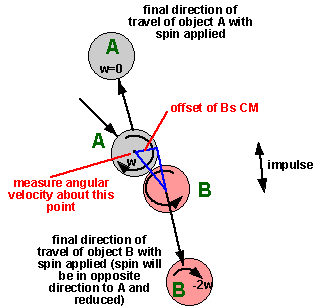# 3D Theory - Example - Snooker

## Collision Detect

We have already discussed the collision between a ball and cushion, here we discuss the collision between balls, how do we detect this collision.

The intersection of two circles (or spheres) is one of the easiest collisions to detect because the shape is simple and it is independent of orientation. The circles are intersecting if the distance between the centres is less than twice the radius. By Pythagoras this is:

(x2-x1)2 + (y2-y1)2 < (2*r)2

where:

• x1,x2 = the horizontal coordinates of the centre of the balls.
• y1,y2 = the vertical coordinates of the centre of the balls.
• r = the radius of the balls.## Collision Response

When the balls collide an impulse will be transferred between the balls, this is a large instantaneous force.

At first sight this seems relatively simple, imagine that the cue ball (on the right) is moving with simple rolling motion, its angular momentum is therefore related to its linear motion.It then hits the stationary object ball and an impulse is transferred between them.After the collision the cue ball is stationary and the object ball moves to the left with rolling motion.Since the object ball is moving in the same way as the object ball was then both linear and angular momentum are conserved then there would appear to be no problem.

However I think its worth investigating this more carefully. How is the angular momentum transferred between the balls? To transfer angular momentum in a collision we need two impulses which are not in line. However the tenancy would be to put backspin rather than forward spin on the object ball, for instance, if the cue ball is spinning in an anti-clockwise direction and is in contact with the object ball then it would tend to turn the object ball in a clockwise direction.So why doesn't this happen? why does the object ball spin in the same direction even though the one of the faces of the balls will be going up and the other will be going down?

The only explanation I can think of for this is that:

• The friction between two balls must be very low
• The friction between a ball and the cloth of the table must be relatively high.

This would mean that there are two sets of impulses which are not in line, this would cause the torque which stops the cue ball from turning and starts the object ball turning.So we have an impulse between the two balls and perpendicular to the face. Because of the low friction there is no component of impulse along the face even though there is relative movement in this direction.

There is also an impulse along the cloth.

## Collision in Two Dimensions

The situation where we ignore rotation and friction is discussed on this page.

## Side Spin

When we take rotation into account then we need to adjust the direction of the impulse accordingly.## Multiple Collisions

If the objects were all moving then we could assume that the balls are unlikely to hit each other simultaneously. Therefore we could avoid the complexities of multiple collisions by assuming an infinitesimally small time difference between the collisions and treat them as a sequence of individual collisions.

However, in snooker, it often happens that there is a block of touching, stationary balls these are then hit by a moving ball. In some cases, it would not be very accurate to model this as a sequence of individual collisions, so we need to work out how the impulse is distributed in the collision.

If they are in a straight line then the impulse will just travel along the line.If they are not in line then it can get more complicated to calculate how the impulse is distributed.Where I can, I have put links to Amazon for books that are relevant to the subject, click on the appropriate country flag to get more details of the book or to buy it from them.

other games programming books

Commercial Software Shop

Where I can, I have put links to Amazon for commercial software, not directly related to this site, but related to the subject being discussed, click on the appropriate country flag to get more details of the software or to buy it from them.Dark Basic Professional Edition - It is better to get this professional editionThis is a version of basic designed for building games, for example to rotate a cube you might do the following: make object cube 1,100 for x=1 to 360 rotate object 1,x,x,0 next x

other commercial software

This site may have errors. Don't use for critical systems.